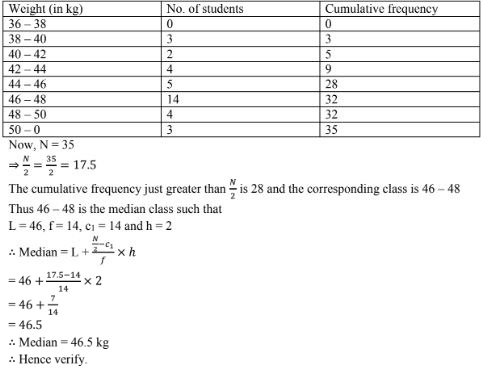# RD Sharma Solutions Chapter 7 Statistics Exercise 7.6 Class 10 Maths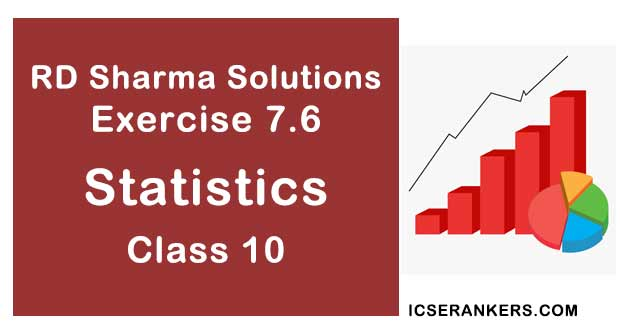Chapter Name RD Sharma Chapter 7 Statistics Book Name RD Sharma Mathematics for Class 10 Other Exercises Exercise 7.1Exercise 7.2Exercise 7.3Exercise 7.4Exercise 7.5 Related Study NCERT Solutions for Class 10 Maths

### Exercise 7.6 Solutions

1. Draw an given by less than method for the following data:

 No. of rooms: 1 2 3 4 5 6 7 8 9 10 No. of houses: 4 9 22 28 24 12 8 6 5 2

Solution
We first prepare the cumulative frequency distribution table by less than method as given be :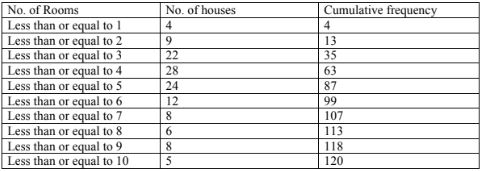Now, we mark the upper class limits along x - axis and cumulative frequency along y - axis.
Thus we plot the point (1,4), (2, 3), (3, 35), (4, 63), (5, 87), (6, 99), (7,107), (8, 113), (9, 118), (10, 120).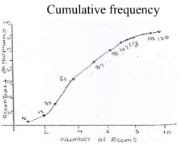2. The marks scored by 750 students in an examination are given in the form of a frequency distribution table:
 Marks No. of students Marks No. of students 600 – 640 16 760 – 800 172 640 – 680 45 800 – 840 59 680 – 720 156 840 – 800 18 720 – 760 284

Prepare a cumulative frequency table by less than method and draw an ogive.

Solution

We first prepare the cumulative frequency table by less than method as given below :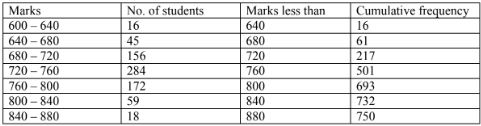Now, we mark the upper class limits along x-axis and cumulative frequency along y-axis on a suitable gear.
Thus, we plot the points (640, 16) (680, 61), (720, 217), (760, 501), (600, 673), (840, 732) and (880, 750).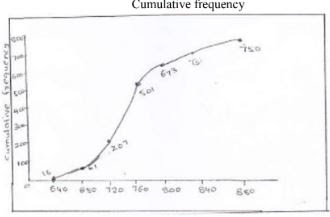3. Draw an ogive to represent the following frequency distribution:
 Class – interval : 0 – 4 5 – 9 10 – 14 15 – 19 20 – 24 No. of students: 2 6 12 5 3

Solution

The given frequency of distribution is not continuous so we first make it continuous and prepare the cumulative frequency distribution as under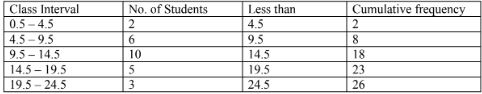Now, we mark the upper class limits along x-axis and cumulative frequency along y-axis.

Thus we plot the points (4, 5, 2), (9, 5, 8), (14, 5, 08), (19, 5, 23) and (24, 5, 26)
Cumulative frequency.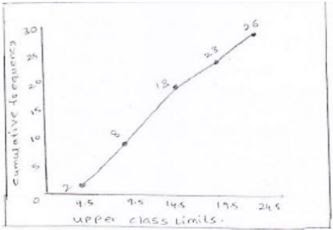4. The monthly profits (in Rs.) of 100 shops are distributed as follows:
 Profits per shop: 0 – 50 50 – 100 100 – 150 150 – 200 200 – 250 250 – 300 No. of shops: 12 18 27 20 17 6

Draw the frequency polygon for it.

Solution

We have,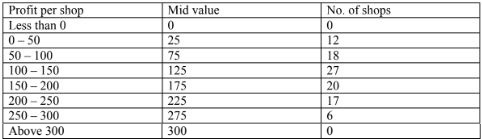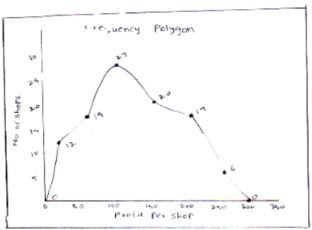5. The following table gives the height of trees:
 Height No. of trees Less than 7 26 Less than 14 57 Less than 21 92 Less than 28 134 Less than 35 216 Less than 42 287 Less than 49 341 Less than 56 360

Draw ‘less than’ ogive and ‘more than’ ogive.

Solution

Less than method,
It is given that,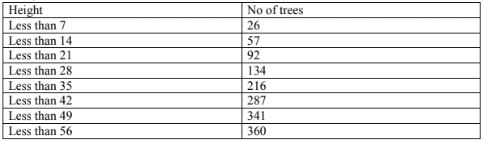Now, we mark the upper class limits along x - axis and cumulative frequency along y - axis.
Thus we plot the points (7, 26) (14, 57) (21, 92) (28, 134) (35, 216) (42, 287) (49, 341) (56, 360)
More than method: we prepare the cf table by more than method as given below: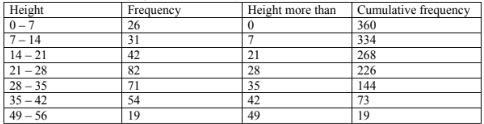Now, we mark on x - axis lower class limits, y - axis cumulative frequency
Thus, we plot graph at(0,360)(7, 334)(14,303)(21, 268)(28,226)(35,144)(42, 73)(49, 19)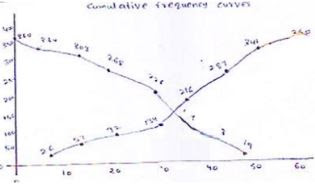6. The annual profits earned by 30 shops of a shopping complex in a locality give rise to the following distribution:
 Profit (in lakhs in Rs) Number of shops(frequency) More than or equal to 5 30 More than or equal to 10 28 More than or equal to 15 16 More than or equal to 20 14 More than or equal to 25 10 More than or equal to 30 7 More than or equal to 35 3

Draw both ogives for the above data and hence obtain the median.

Solution

More than method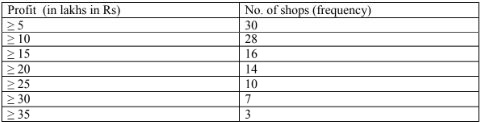Now, we mark on x - axis lower class limits, y - axis cumulative frequency
Thus, we plot the points. (5, 30)(10, 28)(15, 16)(20, 14)(25, 10)(30, 7) and (35, 3)
Less than method.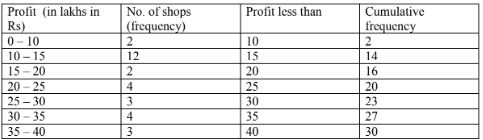Now, we mark the upper class limits along x - axis and cumulative frequency along y - axis.
Thus we plot the points, (10, 2) (15, 14)(20, 16)(25, 20)(30, 23)(35, 27)(40, 30)
We find that the two types of curves intersect of point P from point L it is drawn on x - axis.
The value of a profit corresponding to M is  17.5 lakh, Hence median is 17.5 lakh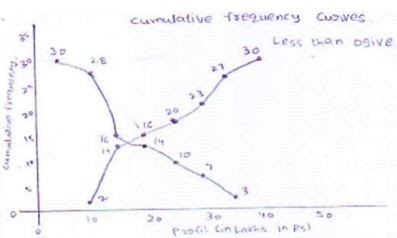7. The following distribution gives the daily income of 50 workers of a factory:
 Daily income (in Rs): 100 – 120 120 – 140 140 – 160 160 – 180 180 – 200 Number of workers: 12 14 8 6 10

Convert the above distribution to a less than type cumula five frequency distribution and draw its ogive.

Solution

We first prepare the cumulative frequency table by less than method as given below.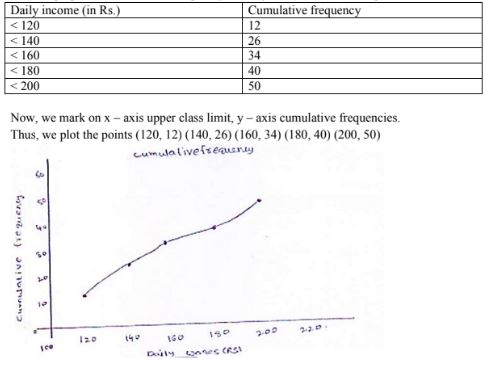8. The following table gives production yield per hectare of wheat of 100 farms of a village:
 Production yield in kg per hectare: 50 – 55 55 – 60 60 – 65 65 – 70 70 – 75 75 – 80 Number of farms: 2 8 12 24 38 16

Draw ‘less than’ ogive and ‘more than’ ogive.

Solution

Less than method:
Cumulative frequency table by less than method.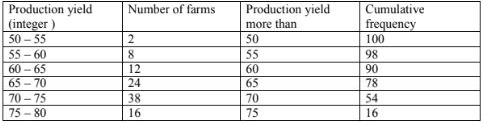Now, we mark on x - axis upper class limit, y - axis cumulative frequencies.
We plot the points(50, 100)(55, 98)(60, 90)(65, 78)(70, 54)(75, 16)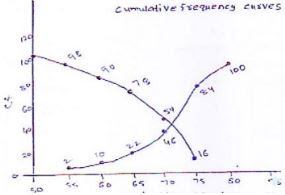9. During the medical check – up of 35 students of a class, their weights were recorded as follows:
 Weight (in kg) No. of students Less than 38 0 Less than 40 3 Less than 42 5 Less than 44 9 Less than 46 14 Less than 48 28 Less than 50 32 Less than 52 35

Draw a less than type ogive for the given data. Hence, obtain the median weight from the graph and verify the result by using the formula.

Solution

Less than method
It is given that
On x - axis upper class limits. Y - axis cf.
We plot the points (38, 0)(40, 3)(42, 5)(44, 9)(46, 4)(48, 28)(50, 32)(52, 35)
More than method: Cf table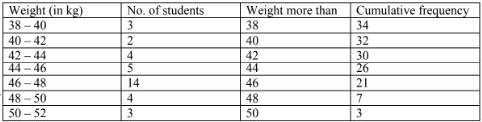x - axis lower class limits on y - axis - cf
We plot the points(38, 35)(40, 32)(42, 30)(44, 26)(46, 21)(48, 7)(50, 3)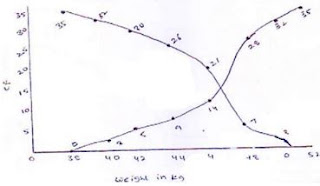We find the two types of curves intersect at a point P. From point P, from P perpendicular PM is draw on x - axis.
The verification,
We have